Home Practice
For learners and parents For teachers and schools
Textbooks
Full catalogue
Pricing SupportLog in

We think you are located in United States. Is this correct?

# 14.7 Complementary events

## 14.7 Complementary events (EMA84)

Complementary set

The complement of a set, $$A$$, is a new set that contains all of the elements that are not in $$A$$. We write the complement of $$A$$ as $$A'$$, or sometimes $$\text{not }\left(A\right)$$.

For an experiment with sample space $$S$$ and an event $$A$$ we can derive some identities for complementary events. Since every element in $$A$$ is not in $$A'$$, we know that complementary events are mutually exclusive.

$A \cap A' = \varnothing$

Since every element in the sample space is either in $$A$$ or in $$A'$$, the union of complementary events covers the sample space.

$A \cup A' = S$

From the previous two identities, we also know that the probabilities of complementary events sum to $$\text{1}$$.

$P\left(A\right) + P\left(A'\right) = P\left(A\cup A'\right) = P\left(S\right) = 1$
temp text

## Worked example 8: Reasoning with Venn diagrams

In a survey $$\text{70}$$ people were questioned about which product they use: A or B or both. The report of the survey shows that $$\text{25}$$ people use product A, $$\text{35}$$ people use product B and $$\text{15}$$ people use neither. Use a Venn diagram to work out how many people:

1. use product A only

2. use product B only

3. use both product A and product B

### Summarise the sizes of the sample space, the event sets, their union and their intersection

• We are told that $$\text{70}$$ people were questioned, so the size of the sample space is $$n\left(S\right) = 70$$.

• We are told that $$\text{25}$$ people use product A, so $$n\left(A\right) = 25$$.

• We are told that $$\text{35}$$ people use product B, so $$n\left(B\right) = 35$$.

• We are told that $$\text{15}$$ people use neither product. This means that $$70 - 15 = 55$$ people use at least one of the two products, so $$n\left(A\cup B\right) = 55$$.

• We are not told how many people use both products, so we have to work out the size of the intersection, $$A \cap B$$, by using the identity for the union of two events:

\begin{align*} P\left(A \cup B\right) & = P\left(A\right) + P\left(B\right) - P\left(A \cap B\right) \\ \frac{n\left(A \cup B\right)}{n\left(S\right)} & = \frac{n\left(A\right)}{n\left(S\right)} + \frac{n\left(B\right)}{n\left(S\right)} - \frac{n\left(A\cap B\right)}{n\left(S\right)} \\ \frac{55}{70} & = \frac{25}{70} + \frac{35}{70} - \frac{n\left(A\cap B\right)}{70} \\ \therefore n\left(A\cap B\right) & = 25 + 35 - 55 \\ & = 5 \end{align*}

### Determine whether the events are mutually exclusive

Since the intersection of the events, $$A \cap B$$, is not empty, the events are not mutually exclusive. This means that their circles should overlap in the Venn diagram.

### Draw the Venn diagram and fill in the numbers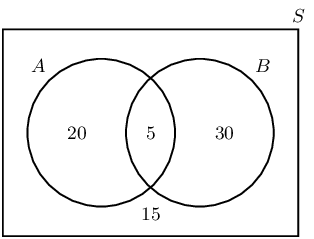1. $$\text{20}$$ people use product A only.

2. $$\text{30}$$ people use product B only.

3. $$\text{5}$$ people use both products.

Textbook Exercise 14.7

A group of learners are given the following Venn diagram: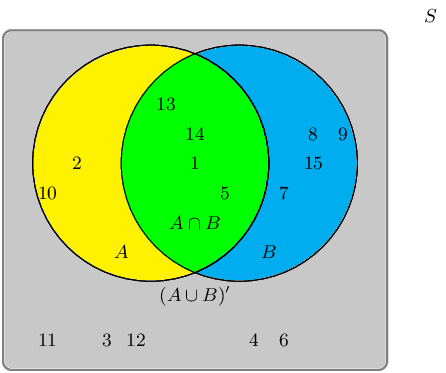The sample space can be described as $$\{ n:n \text{ } \epsilon \text{ } \mathbb{Z}, \text{ } 1 \leq n \leq 15 \}$$.

They are asked to identify the complementary event set of $$B$$, also known as $$B'$$. They get stuck, and you offer to help them find it.

Which of the following sets best describes the event set of $$B'$$?

• $$\{1;5;13;14\}$$
• $$\{2;3;4;6;10;11;12\}$$
• $$\{3;4;6;11;12\}$$

The event set $$B$$ can be shaded as follows: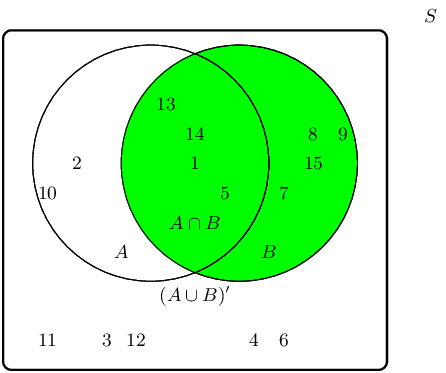The complementary event set $$B'$$ can be shaded as follows: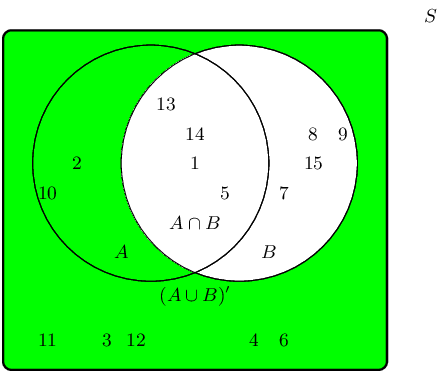Therefore the event set $$\{2;3;4;6;10;11;12\}$$ best describes the complementary event set of $$B$$, also known as $$B'$$.

A group of learners are given the following Venn diagram: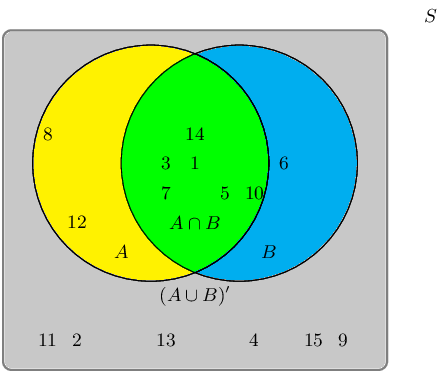The sample space can be described as $$\{ n:n \text{ } \epsilon \text{ } \mathbb{Z}, \text{ } 1 \leq n \leq 15 \}$$.

They are asked to identify the complementary event set of $$(A \cup B)$$, also known as $$(A \cup B)'$$. They get stuck, and you offer to help them find it.

Which of the following sets best describes the event set of $$(A \cup B)'$$?

• $$\{2;4;9;11;13;15\}$$
• $$\{1;3;5;6;7;8;10;12;14\}$$
• $$\{6;8;12\}$$

The event set $$(A \cup B)$$ can be shaded as follows: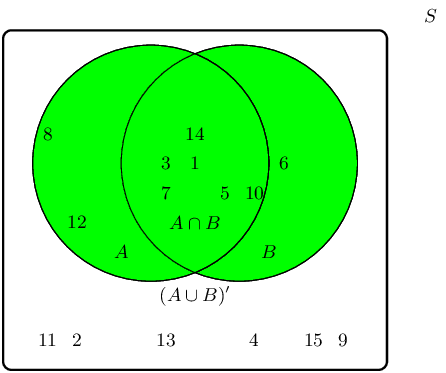The complementary event set $$(A \cup B)'$$ can be shaded as follows: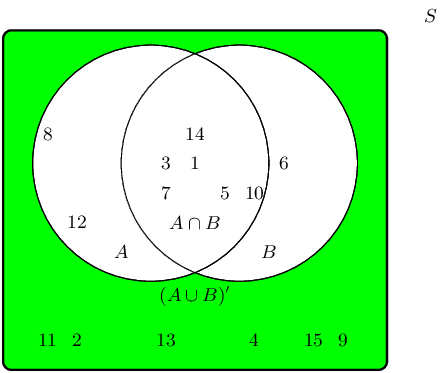Therefore the event set $$\{2;4;9;11;13;15\}$$ best describes the complementary event set of $$(A \cup B)$$, also known as $$(A \cup B)'$$.

Given the following Venn diagram: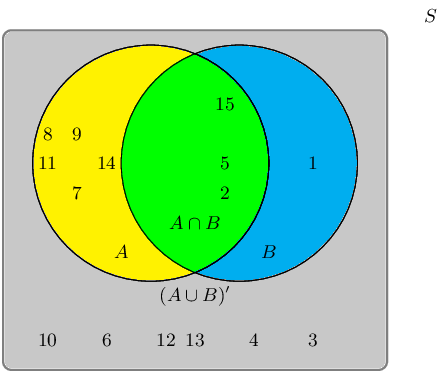The sample space can be described as $$\{ n:n \text{ } \epsilon \text{ } \mathbb{Z}, \text{ } 1 \leq n \leq 15 \}$$.

Are $$(A \cup B)'$$ and $$A \cup B$$ mutually exclusive?

We recall the definition of the term “mutually exclusive”:

Two events are called mutually exclusive if they cannot occur at the same time.

The event set for $$(A \cup B)'$$ is: $$\{3;4;10;12;13\}$$

The event set for $$A \cup B$$ is: $$\{1;2;5;6;7;8;9;11;14;15\}$$

The question we must ask: Can they occur at the same time?

By observing both sets, we can identify the following overlapping event set: $$\{\} \text{ or } \varnothing$$.

Therefore, yes, the event sets $$(A \cup B)'$$ and $$A \cup B$$ are mutually exclusive in this example.

Given the following Venn diagram: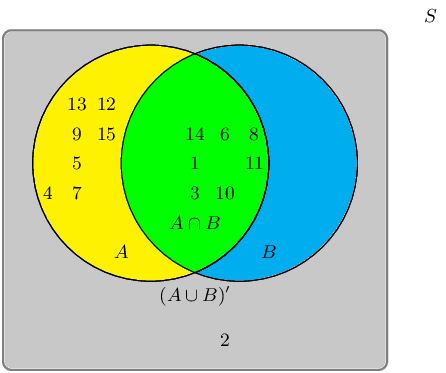The sample space can be described as $$\{ n:n \text{ } \epsilon \text{ } \mathbb{Z}, \text{ } 1 \leq n \leq 15 \}$$.

Are $$A'$$ and $$B'$$ mutually exclusive?

We recall the definition of the term “mutually exclusive”:

Two events are called mutually exclusive if they cannot occur at the same time.

The event set for $$A'$$ is: $$\{2\}$$

The event set for $$B'$$ is: $$\{2;4;5;7;9;12;13;15\}$$

The question we must ask: Can they occur at the same time?

By observing both sets, we can identify the following overlapping event set: $$\{2\}$$

Therefore, no, the event sets $$A'$$ and $$B'$$ are not mutually exclusive in this example.